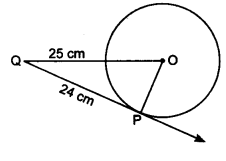# From a point $Q$, the length of the tangent to a circle is $24$ cm and the distance of $Q$ from the centre is $25$ cm. The radius of the circle is(a) 7 cm(b) 12 cm(c) 15 cm(d) 24.5 cm

Given:

From a point $Q$, the length of the tangent to a circle is $24$ cm and the distance of $Q$ from the centre is $25$ cm.

To do:

We have to find the radius of the circle.

Solution:We know that,

The tangent to a circle is perpendicular to the radius through the point of contact.

$\angle OPQ = 90^o$

Therefore, by Pythagoras theorem,

$PQ^2+OP^2 = OQ^2$

$OP^2=OQ^2-PQ^2$

$OP^2 = (25)^2 - (24)^2$

$= 625 - 576$

$= 49$

$OP = \sqrt{49}\ cm$

$OP=7\ cm$

Therefore, the radius of the circle is $7\ cm$.